Checkout JEE MAINS 2022 Question Paper Analysis : Checkout JEE MAINS 2022 Question Paper Analysis :

# Important Questions for Class 11 Chemistry Chapter 8 - Redox Reaction.

Class 11 chemistry important questions with answers are provided here for Chapter 8 Redox Reactions. These important questions are based on the CBSE board curriculum and correspond to the most recent Class 11 chemistry syllabus. By practising these Class 11 important questions, students will be able to quickly review all of the ideas covered in the chapter and prepare for the Class 11 Annual examinations as well as other entrance exams such as NEET and JEE.

Download Class 11 Chemistry Chapter 8 Redox Reactions Important Questions with Answers PDF by clicking the button below.

## Class 11 Redox Reactions Important Questions with Answers

Q1. The reaction

Cl2(g) + 2OH (aq) → CIO (aq) + Cl (aq) + H2O(l)

represents the process of bleaching. Identify and name the species that bleaches the substances due to their oxidising action.

$$\begin{array}{l}Cl_{2}(g) + 2OH^{-}(aq) \to ClO^{-}(aq) + Cl^{-}(aq) + H_{2}O(l)\end{array}$$

Here, Cl2 is both oxidized and reduced in ClO and Cl, respectively. Since Cl cannot act as an oxidising agent (O.A.). Therefore, Cl2 bleaches substances due to oxidising action of hypochlorite ClO ion.

Q2. MnO42- undergoes a disproportionation reaction in an acidic medium but MnO4 does not. Give a reason.

Disproportionation is a redox reaction in which one intermediate oxidation state component transforms into two higher and lower oxidation state compounds.

Manganese’s oxidation states ranging from +2 to +7 in its various compounds. MnO4 has the maximum oxidation state of +7 hence disproportionation is impossible, but MnO42- has a +6 oxidation state, which can be oxidised as well as reduced.

Q3. PbO and PbO₂ react with HCl according to following chemical equations:

2PbO + 4HCl → 2PbCl2 + 2H2O

PbO2 + 4HCI → PbCl2 + Cl2 + 2H2O

Why do these compounds differ in their reactivity?

None of the atoms’ O.N. changes in the first reaction. As a result, it cannot be classified as a redox reaction. Because PbO is a basic oxide that combines with HCl acid, it is an acid-base reaction.

PbO2 is reduced and functions as an oxidising agent in the second process, a redox reaction.

Q4. Nitric acid is an oxidising agent and reacts with PbO, but it does not react with PbO₂. Explain why?

PbO is a basic oxide, and it undergoes a straightforward acid-base reaction with HNO3. In PbO2, on the other hand, lead is in the +4 oxidation state and cannot be further oxidised. As a result, no reaction occurs. PbO2 is thus inactive; only PbO interacts with HNO3.

$$\begin{array}{l}2PbO + 4HNO_{3} \to 2Pb(NO_{3})_{2} + 2H_{2}O\end{array}$$

Q5. Write a balanced chemical equation for the following reactions:

(i) Permanganate ion (MnO4) reacts with sulphur dioxide gas in an acidic medium to produce Mn2+ and hydrogensulphate ion.

(Balance by ion electron method)

(ii) Reaction of liquid hydrazine (N2H4) with chlorate ion (CIO3) in basic medium produces nitric oxide gas and chloride ion in a gaseous state.

(Balance by oxidation number method)

(iii) Dichlorine heptaoxide (Cl2O7) in gaseous state combines with an aqueous solution of hydrogen peroxide in acidic medium to give chlorite ion (ClO2) and oxygen gas.

(Balance by ion electron method)

(i) MnO4 +SO2 → Mn2+ + HSO4(acidic medium)

Balancing by ion-electron method we get:

2 * {MnO4 + 8H+ + 5e → Mn2+ + 4H2O

5 * {SO2 + 2H2O → HSO4 + 3H+ + 2e(ii) N2H4 + ClO3 → NO + Cl (basic medium)

To make the gain and loss of electrons equal, balancing by oxidation number method we get:

3N2H4 + 4ClO3 → 6NO + 4Cl + 6H2O(iii) Cl2O7(g) + H2O2(aq) → 2ClO2 + 3H2O (acidic medium)

2 * {Cl2O7 + 6H+ + 8e → 2ClO2 + 3H2O

8 * {H2O2 → O2 + 2H+ + 2eQ6. Calculate the oxidation number of phosphorus in the following species.

(a) HPO32- and

(b) PO43-

1. Let the oxidation number of phosphorus in HPO32- be x.

H + P + 3O2-

⇒ +1 + x + (-2)*3 = -2

⇒ +1 + x – 6 = -2

⇒ x – 5 = -2

⇒ x = – 2 + 5

⇒ x = +3

Thus, the oxidation number of phosphorus is +3.

1. Let the oxidation number of phosphorus in PO43- be x.

PO43-

⇒ x + 4 * (-2) = -3

⇒ x = -3 + 8 = +5

⇒ x = +5

Thus, the oxidation number of phosphorus is +5.

Q7. Calculate the oxidation number of each sulphur atom in the following compounds:

(a) Na2S2O3

(b) Na2S4O6

(c) Na2SO3

(d) Na2SO4

1. The inorganic chemical sodium thiosulfate (also known as sodium thiosulphate) has the formula Na2S2O3 xH2O.

We can’t find the oxidation number of each sulphur atom using traditional methods.

Structure will provide a clear explanation. One sulphur molecule possesses +6 O.S., whereas the other has -2.1. Let the oxidation number of sulphur in Na2S4O6 be x.

⇒ 2 + 4x – 12 = 0

⇒ 4x = +10

⇒ x = 10/4

⇒ x = +2.5

Thus, the oxidation number of sulphur in Na2S4O6 is +2.5.

(c) Let the oxidation number in Na2SO3 be x.

Na2 S O3

⇒ 2×(+1)+x+(−2)×3 = 0

⇒ 2+x−6 = 0

⇒ x = +4

Thus, the oxidation number of sulphur in Na2SO3 is +4.

(d) Let the oxidation number of sulphur in Na2SO4 be x.

⇒ 2×(+1)+x+(−2)×4 = 0

⇒ 2+x−8 = 0

⇒ x = +6

Thus, the oxidation number of sulphur in Na2SO4 is +6.

Q8. Balance the following equations by the oxidation number method.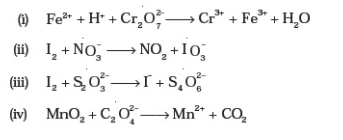(i) Fe2+ + Cr2O72- + H+ → Fe3+ + Cr3+ + H2O

The chromium oxidation number drops from +6 in Cr2O72 to +3 in Cr3+. In Cr2O72- to Cr3+, the total drop for two chromium atoms is 6. Iron’s oxidation number, on the other hand, rises from +2 (in Fe2+) to +3 (in Fe3+).

Multiply Fe2+ by 6 and Cr2O72- by 1 to balance the increase and reduction in oxidation numbers. Then there’s,

6Fe2+ + Cr2O72- + H+ → Fe3+ + Cr3+ + H2O

On both sides of the reaction, Fe and Cr atoms must be balanced.

6Fe2+ + Cr2O72- + H+ → 6Fe3+ + 2Cr3+ + H2O

Multiply H2O by 7 to balance O atoms.

6Fe2+ + Cr2O72- + H+ → 6Fe3+ + 2Cr3+ + 7H2O

Multiply H+ by 14 to balance H-atoms.

6Fe2+ + Cr2O72- + 14H+ → 6Fe3+ + 2Cr3+ + 7H2O

(ii) The given skelton equation is: I2 + NO3 → NO2 + IO3

Let us find the oxidation number of both I and N.

LHS,

I2

⇒ 2x = 0

⇒ x = 0

IO3

⇒ x + 3 (−2) = −1

⇒ x = +5

For one atom of I, the oxidation number rises by 5. It’s 10 for two atoms.

NO3

⇒ x + 3 (−2) = −1

⇒ x = +5

NO2

⇒ x + 2 (−2) = 0

⇒ x = +4

As a result, the oxidation number drops by 1.

Cross multiplying the oxidation number and writing the equation,

I2 + 10NO3 →NO2 + IO3−

Balancing the RHS side I and N,

I2 + 10NO3 →10NO2 + 2 IO3

Now, balancing the the oxygen on both the sides by adding H2O,

I2 + 10NO3 →10 NO2 + 2IO3 + 4H2O

Balance the hydrogen by adding H+,

I2 + 10NO3 + 8H+ →10 NO2 + 2IO3 + 4H2O

Hence, the balanced equation is,

I2 + 10NO3 + 8H+ →10NO2 + 2IO3 + 4H2O

(iii) The unbalanced redox reaction is I2 + S2O32- → I + S4O62-

Balancing all atoms other than H and O, we get

I2 + 2S2O32- → 2I + S4O62-

I changes its oxidation number from 0 to -1. The oxidation number of one I atom changes by one. For 2 I atoms, the total change in oxidation number is 2.

The oxidation number of S increases from 2 to 2.5, representing a 0.5 increase in the oxidation number of one S atom.

For four S atoms, the total change in oxidation number is 2.

Increases in oxidation number are counterbalanced by decreases in oxidation number.

The atoms of O are in a state of equilibrium. This is the chemical equation that is balanced.

(iv) MnO2 + C2O42- → Mn2+ + CO2

Balancing all atoms, we get: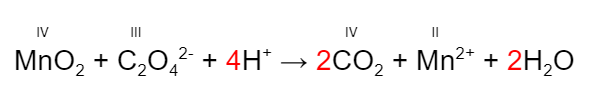The following is an example of an oxidation-reduction (redox) reaction:

MnIV + 2e → MnII (reduction)

2CIII – 2e → 2CIV (oxidation)

C2O42- is a reducing agent, while MnO2 is an oxidising agent.

Q9. Identify the redox reactions out of the following reactions and identify the oxidising and reducing agents in them.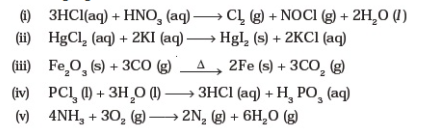(i) Writing the O.N. of on each atom, we get:

3HCl + HNO3 → Cl2 + NOCl + 2H2O

Cl’s O.N. goes from -1 (in HCl) to 0 in this case (in Cl2). As a result of the oxidation of Cl, HCl is used as a reducing agent.

HNO3 functions as an oxidising agent because the O.N. of N reduces from +5 (in HNO3) to +3 (in NOCl).

Thus, reaction (i) is, therefore, a redox reaction.

(ii) HgCl2 + 2Kl → Hgl + 2KCl

There is no change in O.N. of any of the atoms, hence this is not a redox reaction.

(iii) Fe2O3 + 3CO → 2Fe +3CO2

Fe2O3 works as an oxidising agent because the O.N. of Fe falls from +3 (in Fe2O3) to 0 (in Fe).

CO works as a reducing agent because the O.N. of C increases from +2 (in CO) to +4 (in CO2). As a result, we have a redox reaction.

(iv) PCl3 + 3H2O → 3HCl + H2PO3

There is no change in O.N. of any of the atoms, hence it is not a redox reaction.

(v) 4NH3 + 3O2 → 2N2 + 6H2O

Because the O.N. of N in N2 grows from -3 to 0, NH3 functions as a reducing agent.

Furthermore, because the O.N. of O drops from 0 to O2 to -2 in H2O, O2 functions as an oxidizing agent. As a result, this is a redox reaction.

Q10. Balance the following ionic equations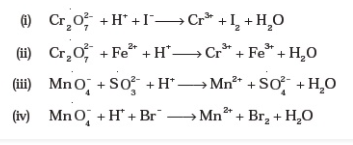Balanced equations of given unbalanced equations:

(i)

$$\begin{array}{l}Cr_{2}O_{7}^{-2} + 6I^{-} + 14H^{+} \rightleftharpoons 2Cr^{+3} + 3I_{2} + 7H_{2}O\end{array}$$

(ii)

$$\begin{array}{l}Cr_{2}O_{7}^{-2} + 6Fe^{+2} + 14H^{+} \rightleftharpoons 2Cr^{+3} + 6Fe^{+3} + 7H_{2}O\end{array}$$

(iii)

$$\begin{array}{l}2MnO_{4}^{-} + 5SO_{3}^{-2} + 6H^{+} \rightleftharpoons 2Mn^{+2} + 5SO_{4}^{-2} + 3H_{2}O\end{array}$$

(iv)

$$\begin{array}{l}2MnO_{4}^{-} + 10Br^{-} + 16H^{+} \rightleftharpoons 2Mn^{+2} + 5Br_{2} + 8H_{2}O\end{array}$$

Q1. Explain redox reactions on the basis of electron transfer. Give suitable examples.

An oxidation-reduction (redox) reaction is a chemical reaction in which electrons are transferred between two substances. Any chemical reaction in which a molecule, atom, or ion’s oxidation number changes by acquiring or losing an electron is known as an oxidation-reduction reaction.

As we know, the reactions,

2Na(s) + Cl2(g) → 2NaCl(s)

4Na(s) + O2(g) → 2Na2(s)

because each of these reactions involves the addition of oxygen or a more electronegative element to sodium, they are redox reactions.

At the same time, chlorine and oxygen are being depleted, while sodium, an electropositive element, has been added. We also know that sodium chloride and sodium oxide are ionic compounds and are perhaps better expressed as Na+Cl(s) and Na2+O2, respectively, based on our understanding of chemical bonding(s). The development of charges on the species created leads us to recast the preceding reaction: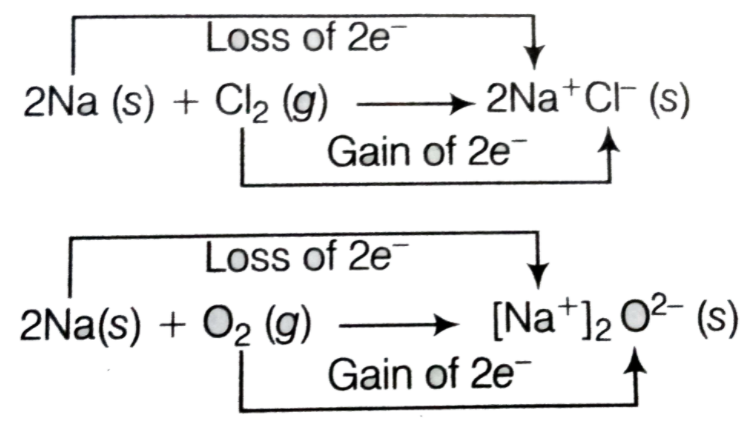Each of the above processes can be thought of as two different phases, one involving electron loss and the other involving electron gain. We can expound on one of these, say sodium chloride production, as an example.

2Na(s) → 2Na+(g) + 2e

Cl2 + 2e → 2Cl(g)

Each of the preceding steps is referred to as a half-reaction since it clearly demonstrates the involvement of electrons. The total reaction is equal to the sum of the half-reactions:

2Na(s) + Cl2(g) → 2Na + Cl(s) or 2NaCl(s)

The above reactions indicate that 50% of the reactions involving electron loss are oxidation reactions. Similarly, half-reactions involving electron gain are referred to as reduction reactions.

The basic operations of life, such as photosynthesis, respiration, combustion, and corrosion or rusting, are redox reactions.

Q2. On the basis of standard electrode potential values, suggest which of the following reactions would take place? (Consult the book for Eo value).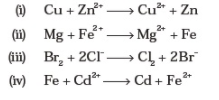The cell’s net cell EMF determines whether or not a reaction will occur. The formula is as follows:

$$\begin{array}{l}E^{o}_{cell} = E^{o}_{cathode} – E^{o}_{anode}\end{array}$$

Option (ii) clearly shows that the reaction can occur because Mg has a lower Ecirc cell value. As a result, Mg is oxidised by losing an electron, whereas iron is reduced by gaining one.

$$\begin{array}{l}Mg + Fe^{2+} \to Mg^{2+} + Fe\end{array}$$

We can state that Fe goes through reduction and Mg goes through oxidation.

Taking the Eo values,

Eocathode = -0.44V

Eoanode = -2.36V

Eocell = -0.44 – (-2.36)V

Eocell = +1.92V

Q3. Why does fluorine not show a disproportionation reaction?

Disproportionation is a redox reaction in which one intermediate oxidation state component transforms into two higher and lower oxidation state compounds.

The element must be in at least three oxidation states for such a redox reaction to occur. As a result, that element is in the intermediate state during the disproportionation reaction and can transition to both higher and lower oxidation states.

Fluorine is the most electronegative and oxidising element of all the halogens, and it is also the smallest.

It doesn’t have a positive oxidation state (only one) and doesn’t go through the disproportionation reaction.

Q4. Find out the oxidation number of chlorine in the following compounds and arrange them in increasing order of oxidation number of chlorine.

NaClO4, NaClO3, NaCIO, KCIO2, Cl2O7, ClO3, Cl2O, NaCl, Cl2, CIO2.

Which oxidation state is not present in any of the above compounds?

Considering oxidation number of chlorine is ‘x’, then 1 + x + 4 * (-2) = 0

∴ x – 7 = 0

∴ x = +7

Na(+1)Cl(+7)O4(-2)

Here, oxidation number of chlorine is +7.

Following the above method,

Na(+1)Cl(+1)O3(-2) Oxidation number of chlorine = +5

Na(+1)Cl(+1)O(-2) Oxidation number of chlorine = +1

K(+1)Cl(+3)O2(-2) Oxidation number of chlorine = +3

Cl2(+7)O7(-2) Oxidation number of chlorine = +7

Cl(+6)O3(-2) Oxidation number of chlorine = +6

Cl2(+1)O(-2) Oxidation number of chlorine = +1

Na(+1)Cl(-1) Oxidation number of chlorine = -1

Cl2 Oxidation number of chlorine = 0

Cl(+4)O2(-2) Oxidation number of chlorine = +4

The following compounds are listed in increasing order of chlorine oxidation number:

NaCl, Cl2, Cl2O, NaCIO, KCIO2, CIO2, NaClO3, ClO3, Cl2O7, NaClO4.

Q5. Which method can be used to find out the strength of the reductant/oxidant in a solution? Explain with an example.

When a reductant (reducing agent) or oxidant (oxidising agent) is linked in a solution using a cell, the relative electrode potential can be measured. The element under discussion can be used as an electrode in a conventional cell with a known electrode potential. The electrode of the given species works as a reductant if it is positive and an oxidant if it is negative.

For example, we want to test Fe3+/Fe with a Standard Hydrogen electrode (SHE). For Fe and H, the half-cell reaction is as follows:

H+ + e → H2Eo = 0.0V

Fe3+ + e → Fe2+Eo = 0.77

With SHE, any element that needs to be evaluated is used as an electrode. The element’s potential is defined as the quantity of emf it generates in the cell.

Eocell = Eocathode – Eoanode

Eocell= 0 – Eoanode

Eocell = 0 – 0.77

Eocell= -0.77

In comparison to hydrogen, the Fe3+ ion has a higher tendency to undergo reduction. As a result, the previously assumed Fe anode configuration can be reversed, and the strength of Fe as a reductant can be established. As a result, the oxidant’s strength can be determined.

Test your knowledge on Important Questions Class 11 Chemistry Chapter 8 Redox Reaction